Having trouble getting all the keys in Uncharted: Fortune Hunter? Don't worry, we've got your back. Follow these guides and you'll have them all in no time flat.

### Level 21

First move here.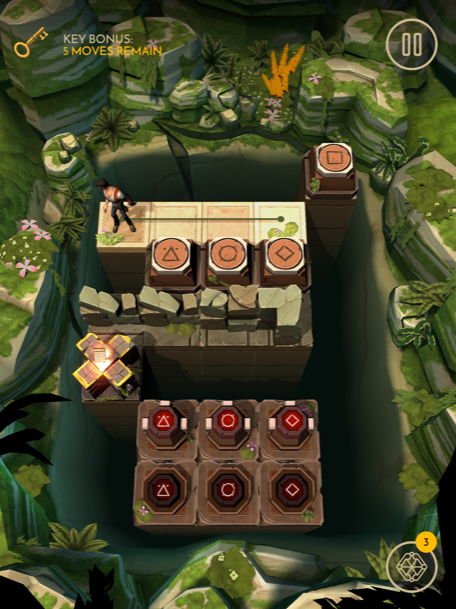Tap the switch, then move here.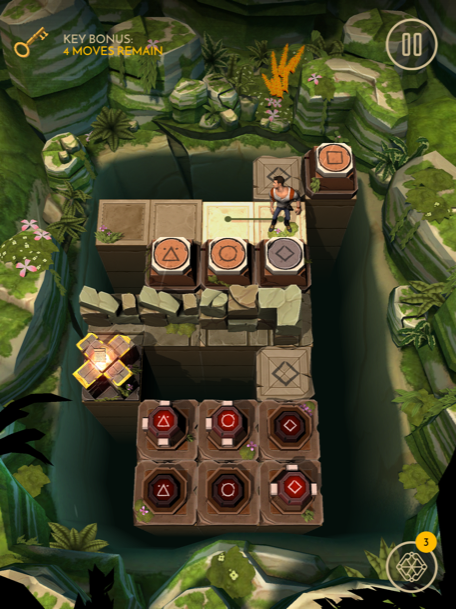Tap the switch and move here.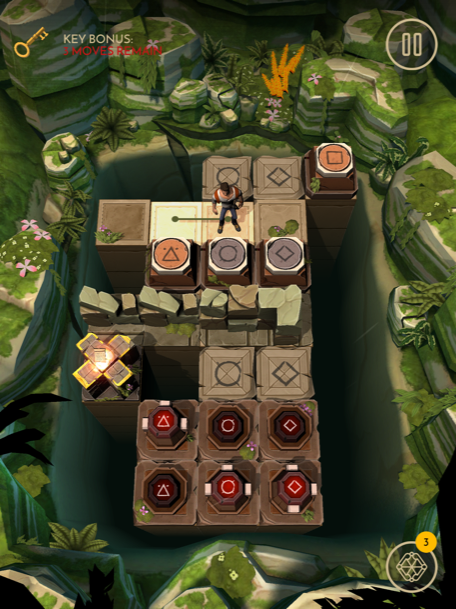Tap the switch, then follow this path.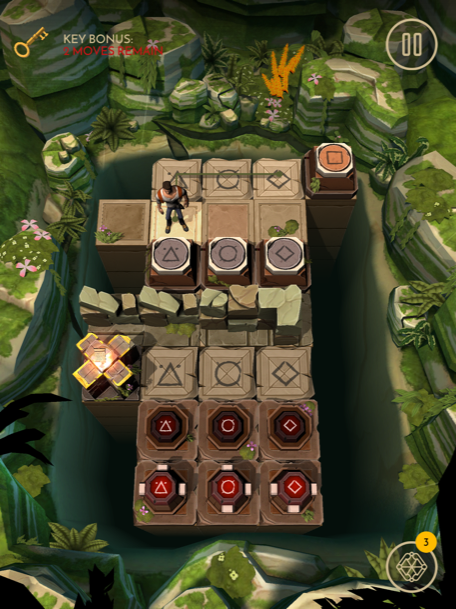Tap the switch, then follow this path to the treasure.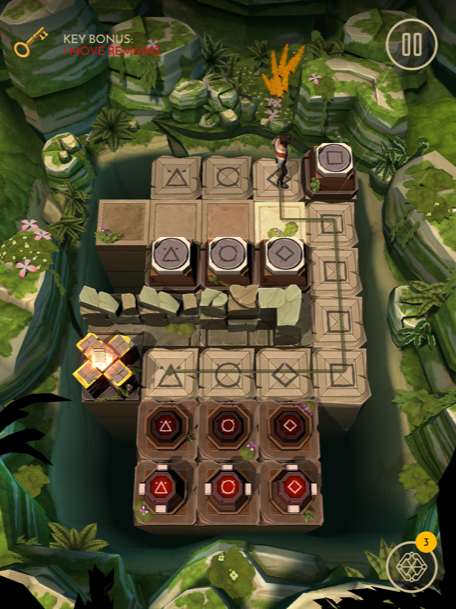### Level 22

First move here and pick up the bomb.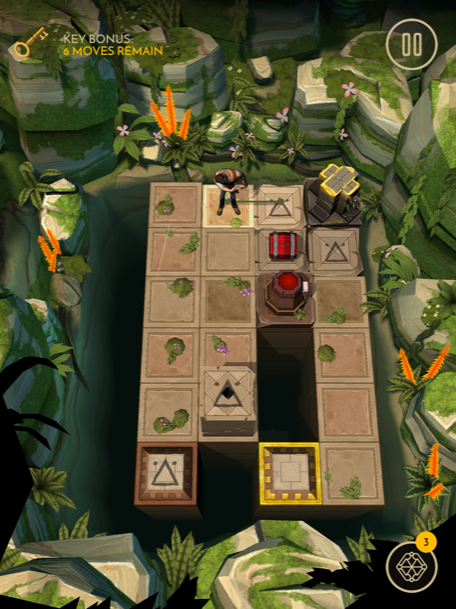Now follow this path and drop the bomb on the switch.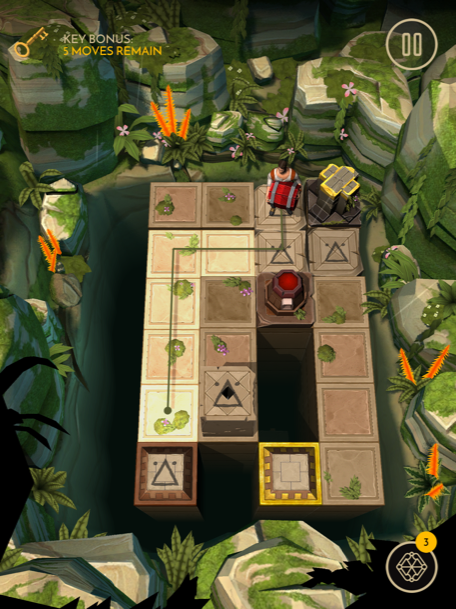Follow this path, then tap on the block.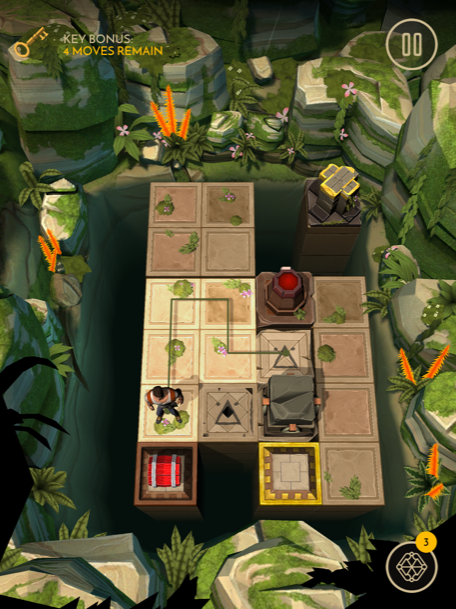Push the block onto the switch.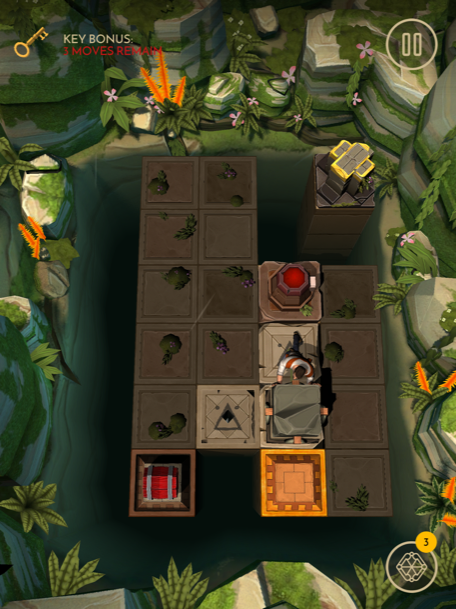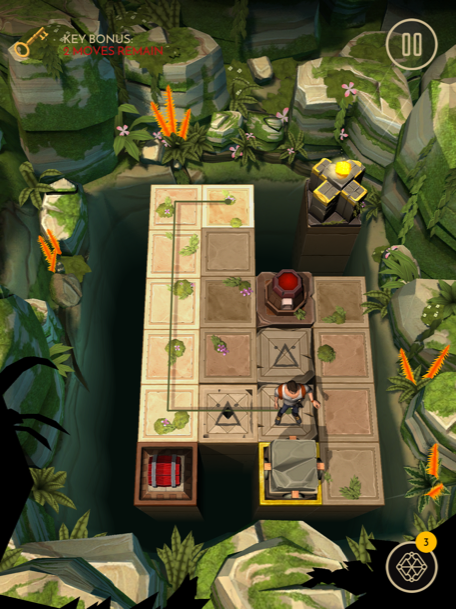Shoot the bomb, then follow this path to get the treasure.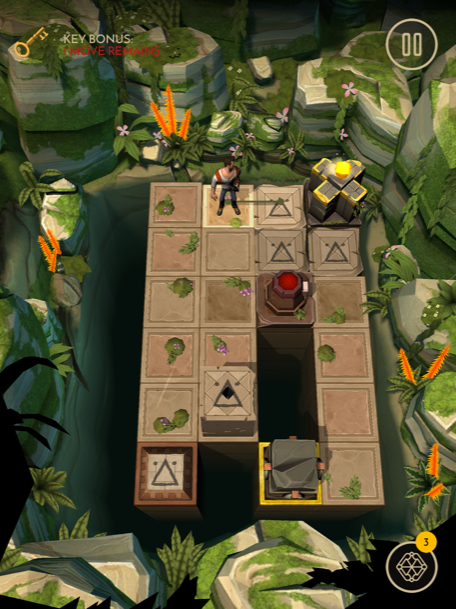### Level 23

First tap the block, then push it here.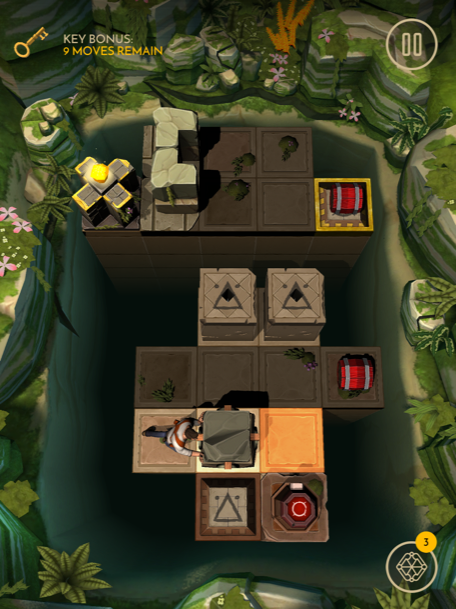Next move here and grab the bomb.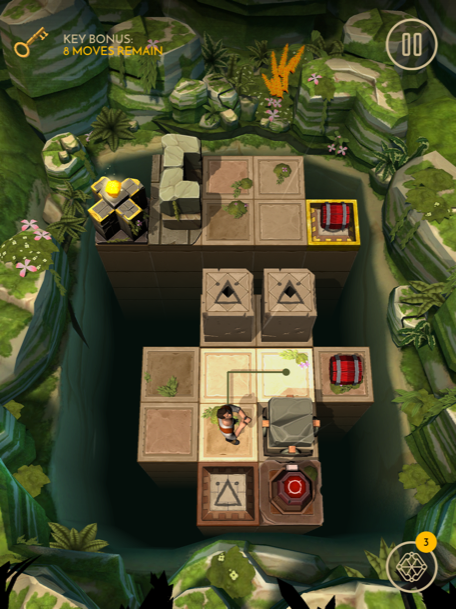Move here and drop the bomb on the switch.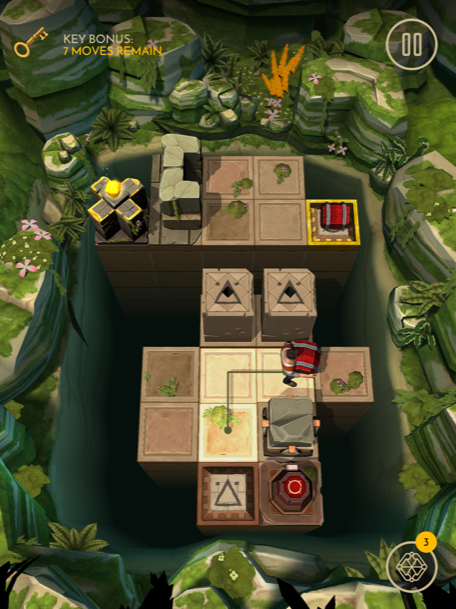Now move here.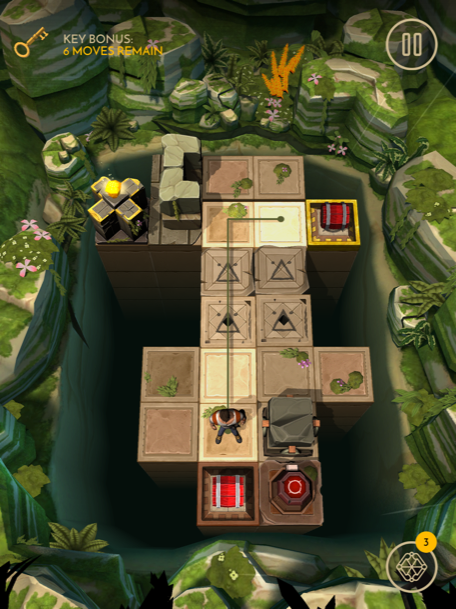Pick up the bomb, place it behind you, then move here.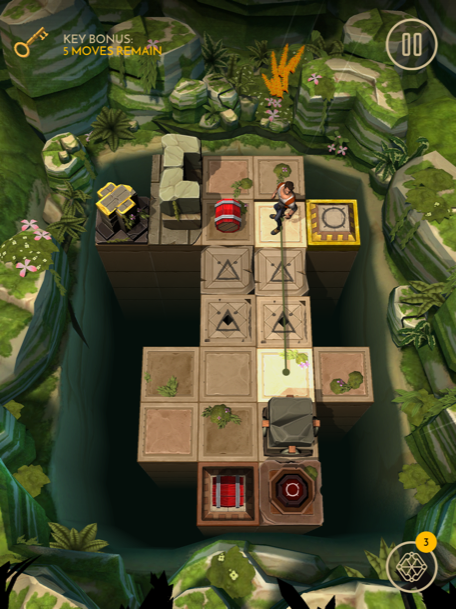Shoot the bomb at the top of the level, tap the block, then drag it here.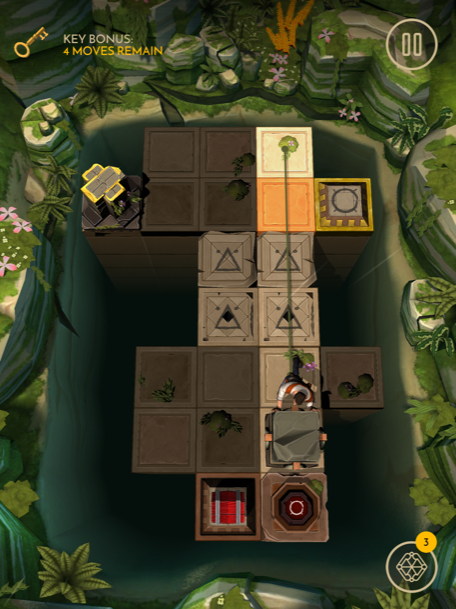Move here, then tap the block.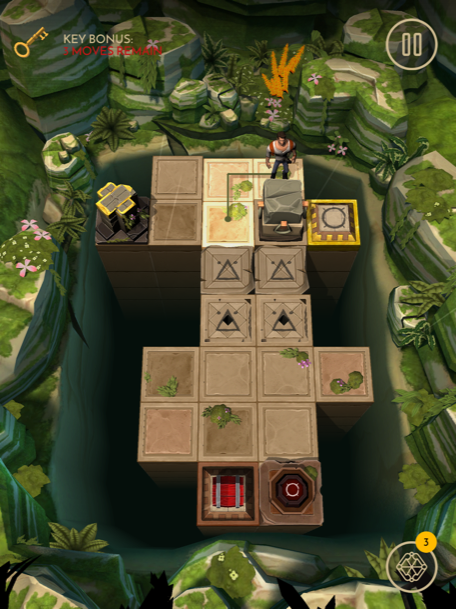Push the block onto the switch.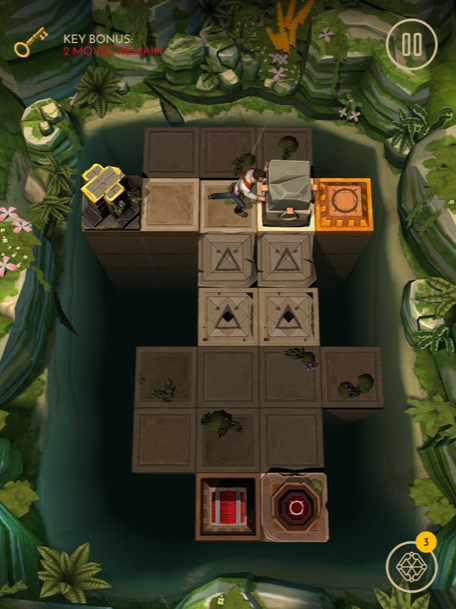Now follow this path and grab the treasure.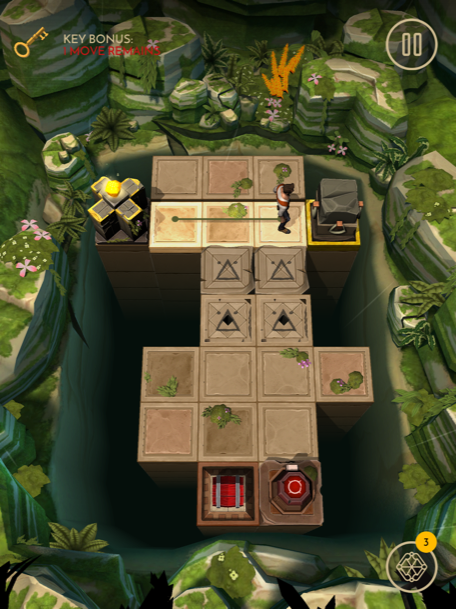### Level 24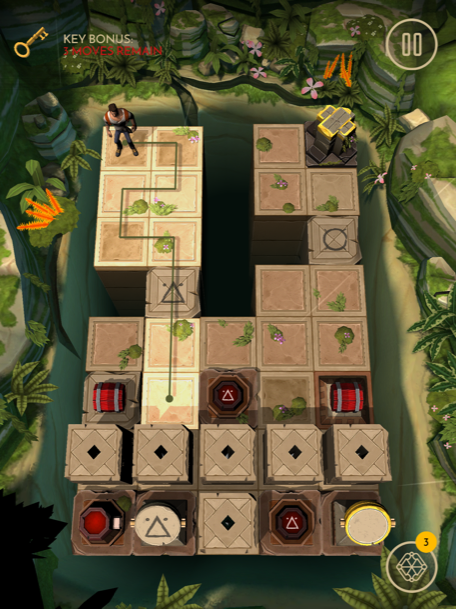Grab the bomb, then move here.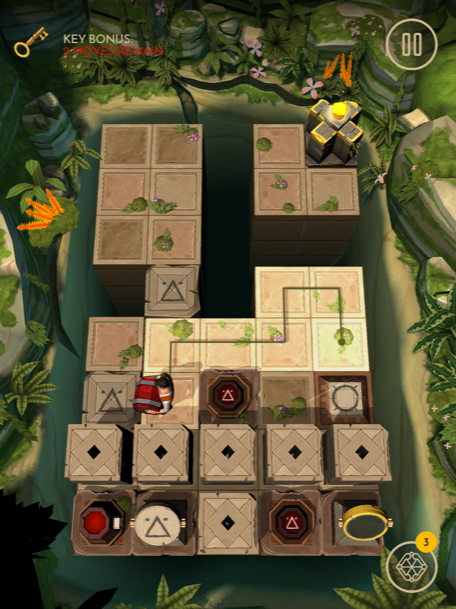Place the bomb on the switch, then follow this path to the treasure.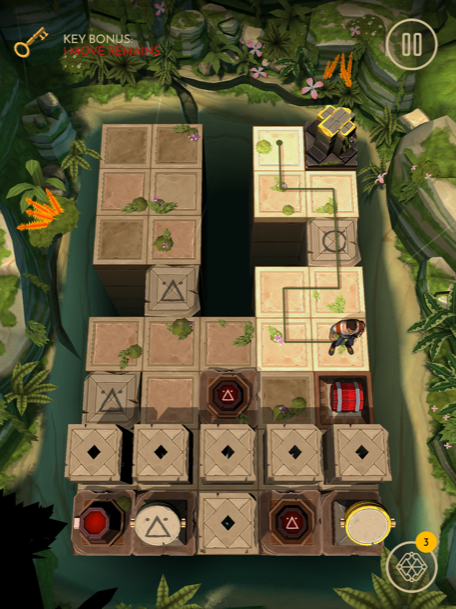### Level 25

Move here and push the button.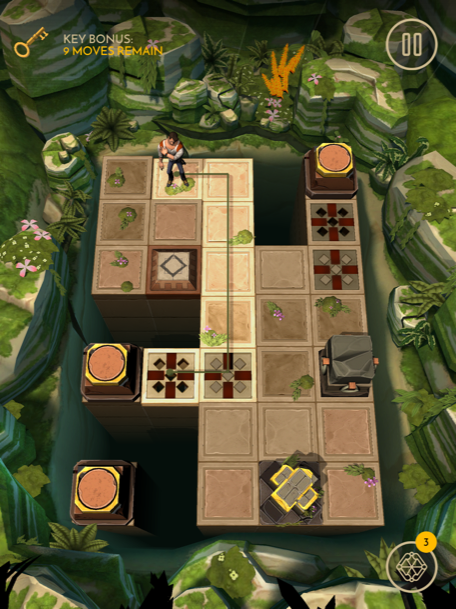Now move here and push the button.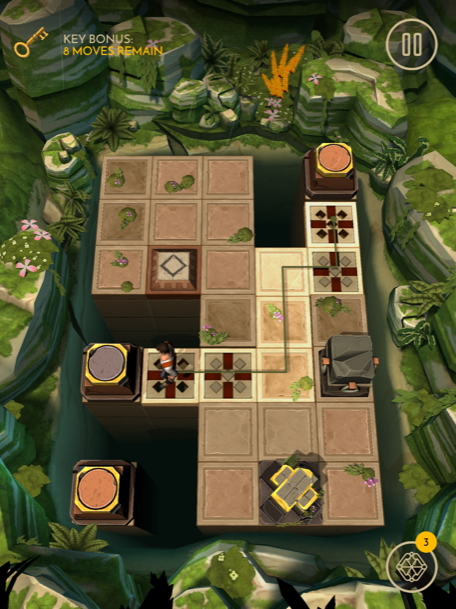Move here and tap the block.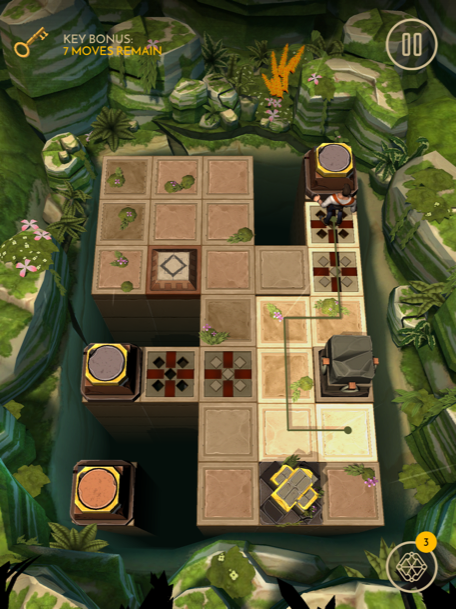Push the block here.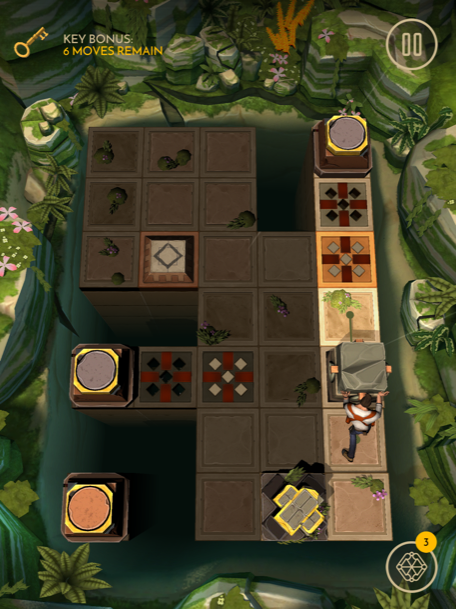Now move here and tap the block.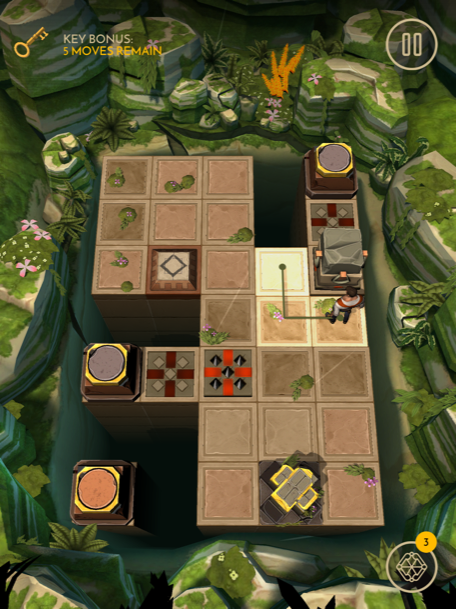Drag the block here.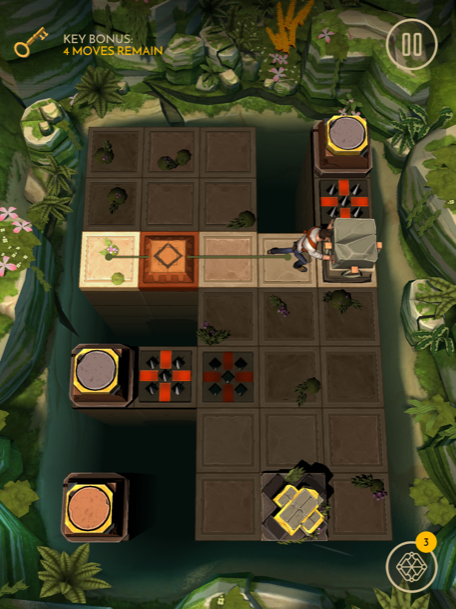Follow this path and push the switch.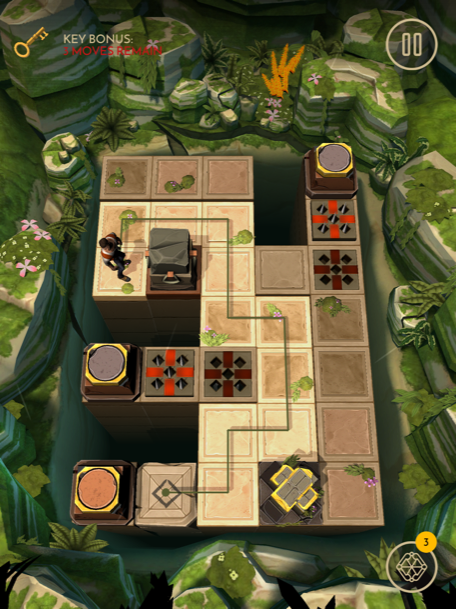Now head here and grab the treasure.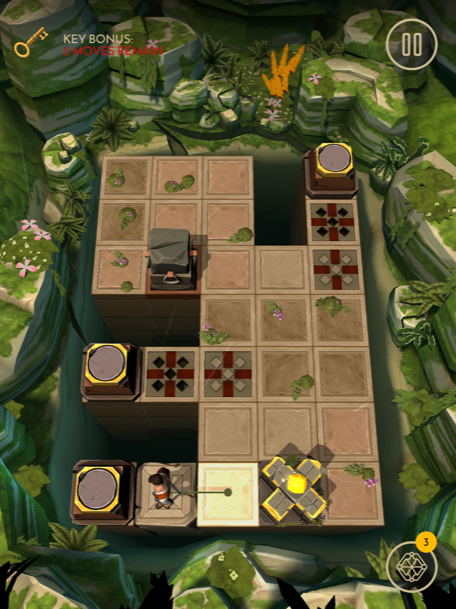### Level 26

First move here, then tap the block.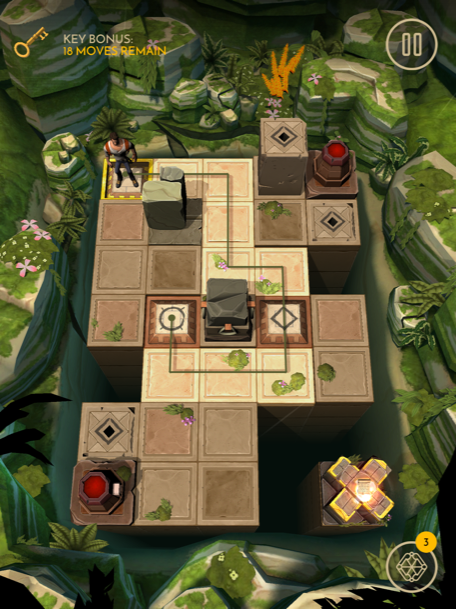Push the block here.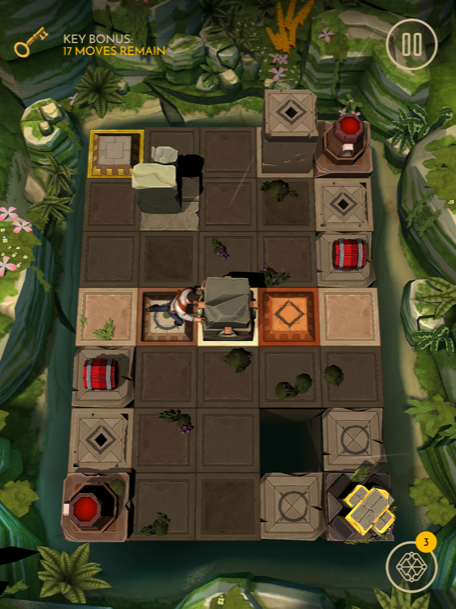Now tap the block, and drag it here.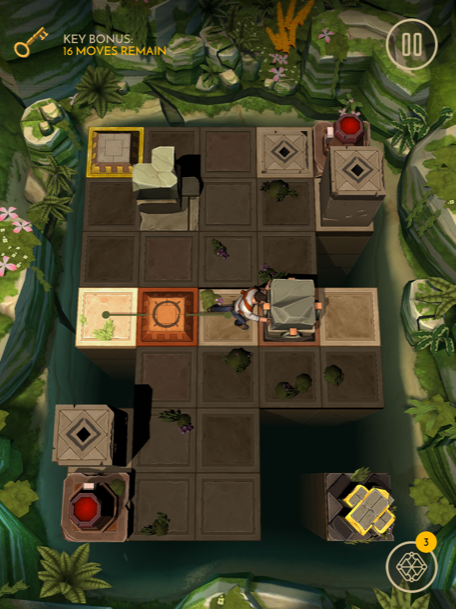Pick up the bomb, place it above you, then tap the block and push it here.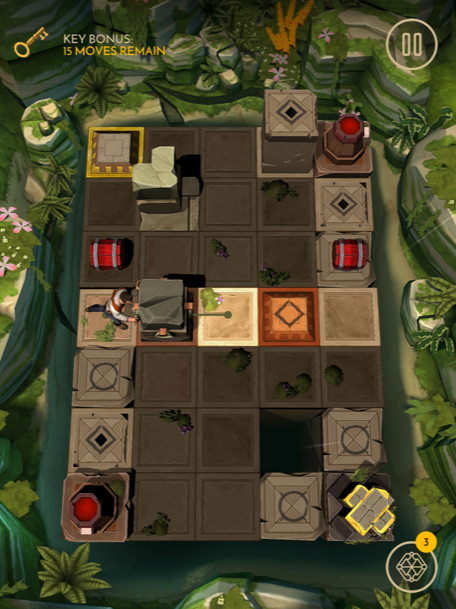Now move here and pick up the bomb.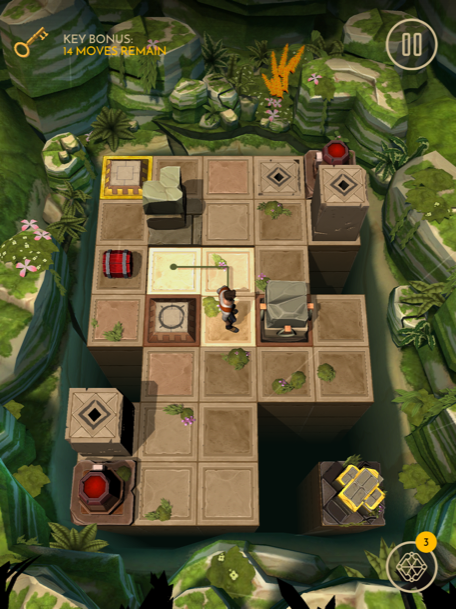Place the bomb on the switch, then move here.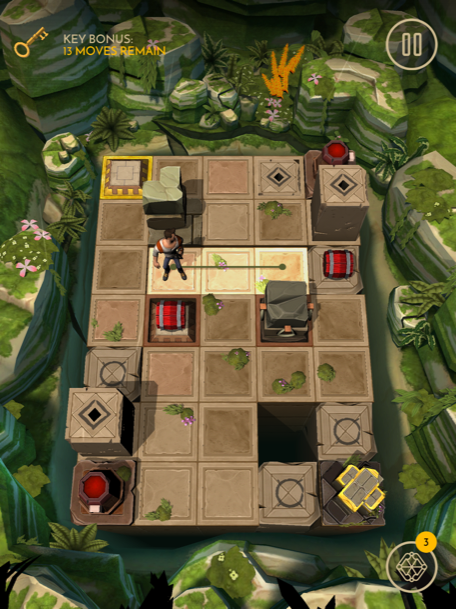Put the bomb above you, then move here.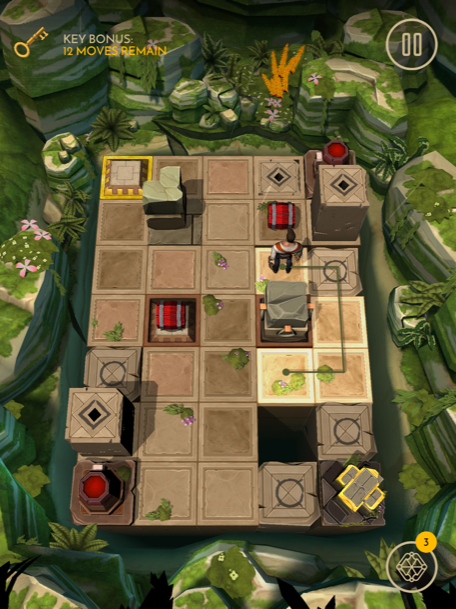Tap the block and push it here.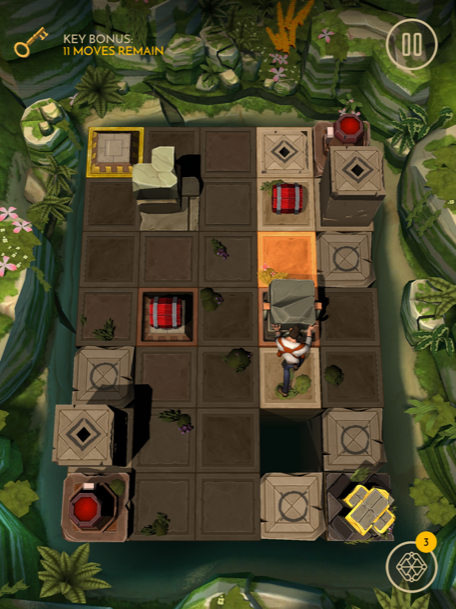Move here and tap the block.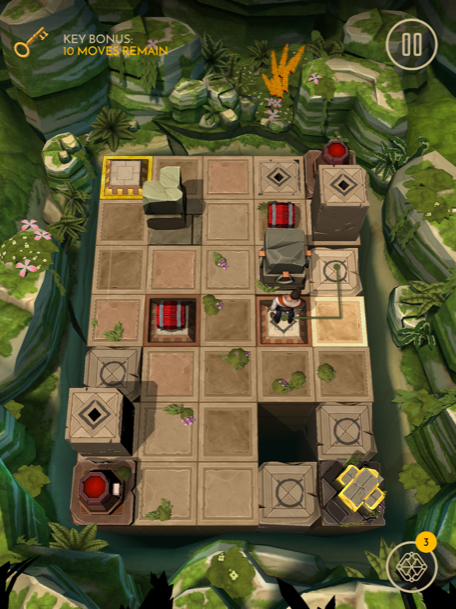Tap the block and push it here.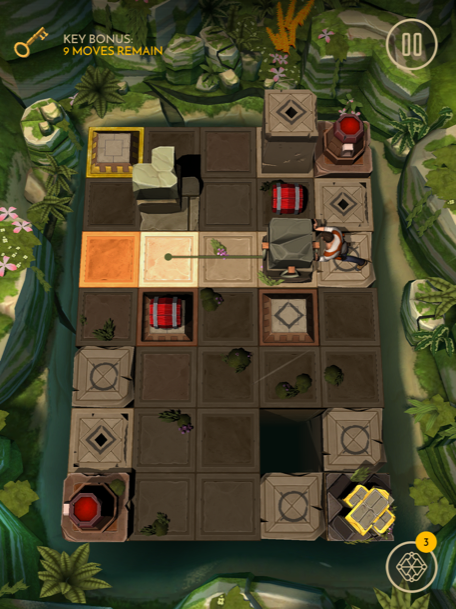Move here, pick up the bomb, and place it on the switch.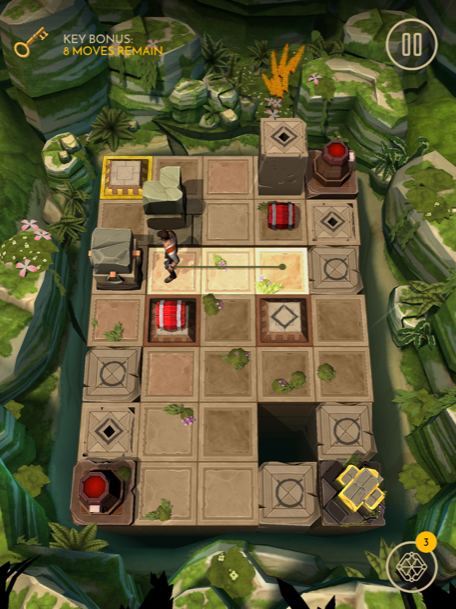Now move here and tap the block.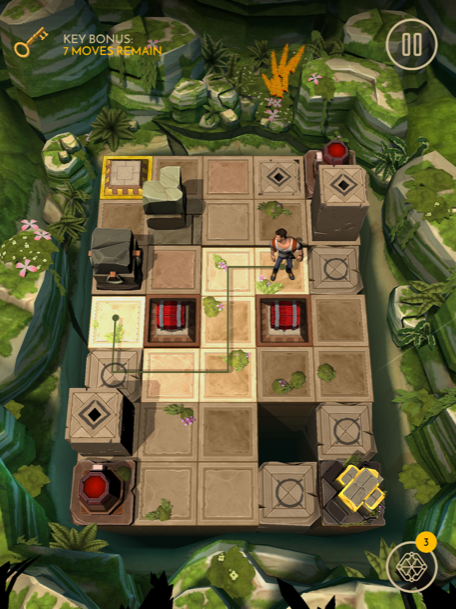Push the block here.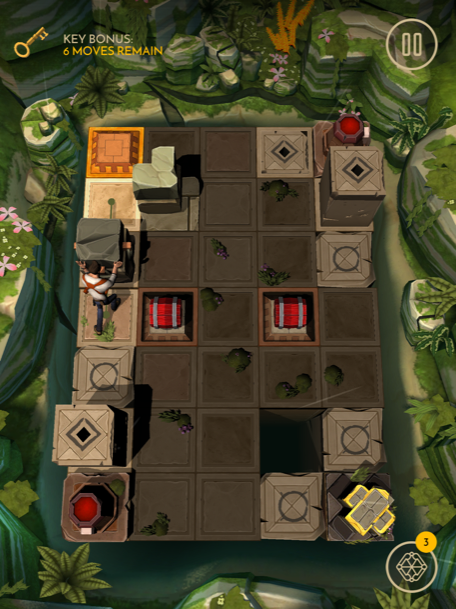Now follow this path to the treasure.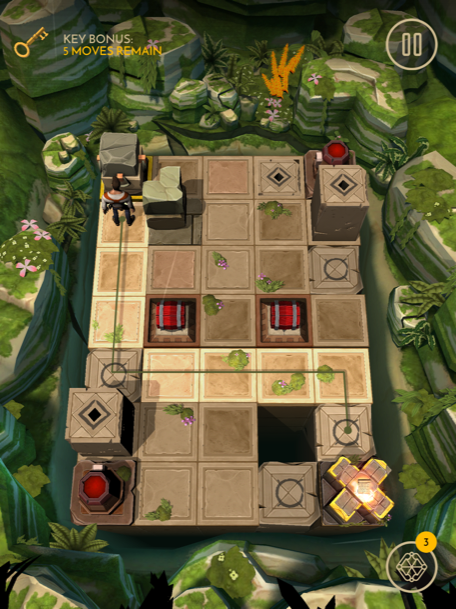### Level 27

Move here and shoot the plate.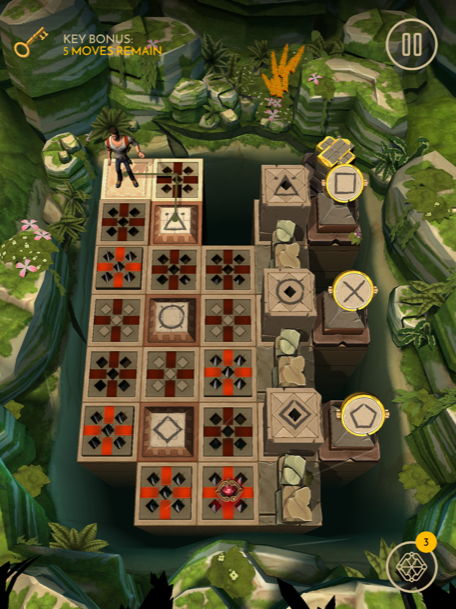Move here and shoot the plate.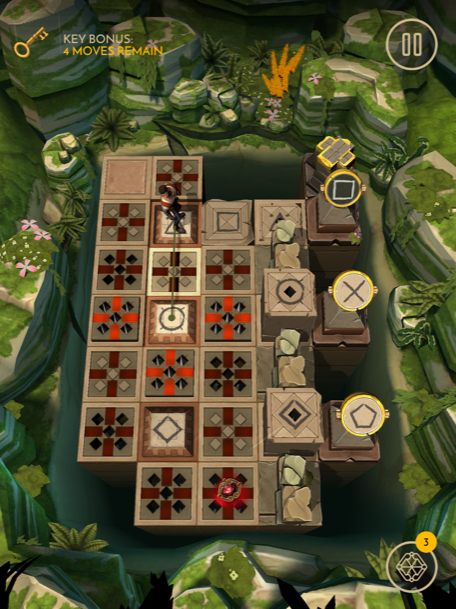Move here and shoot the plate.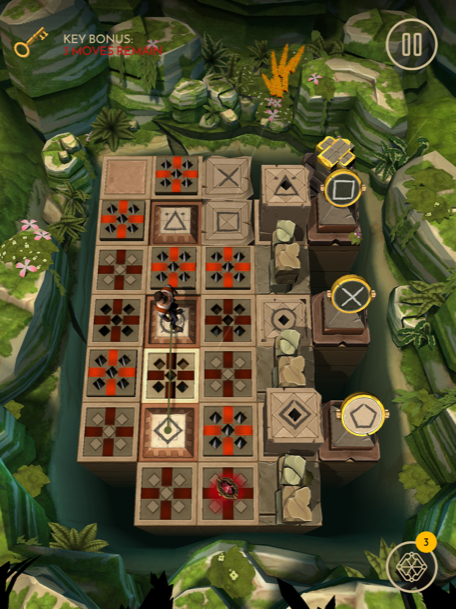Now follow this path and grab the treasure.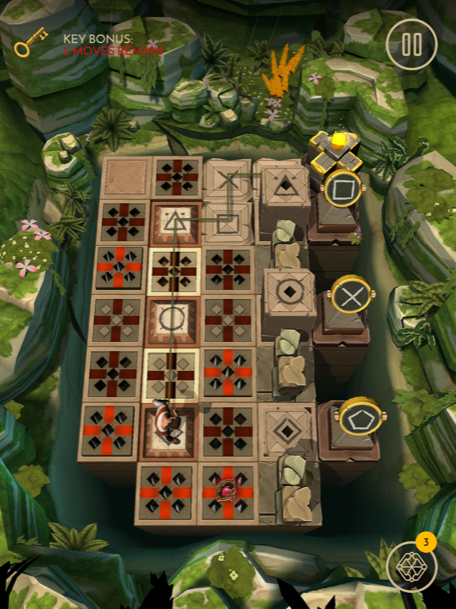### Level 28

First move here.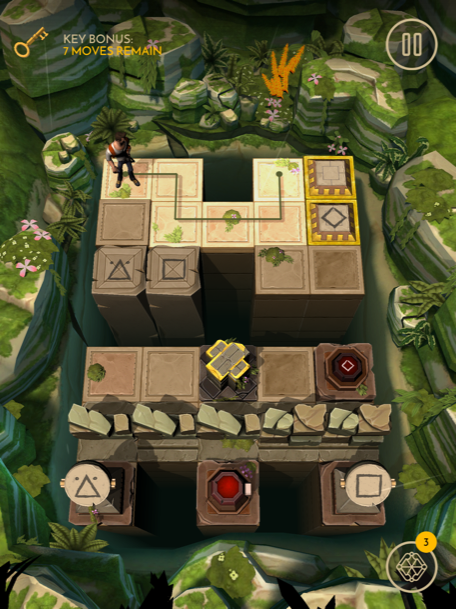Pick up the block, place it on the switch, then move here.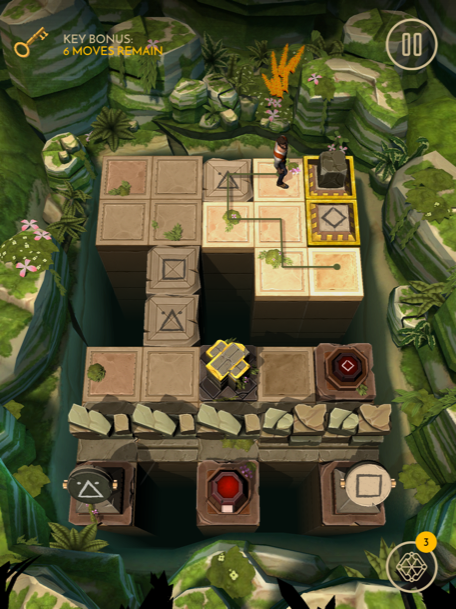Pick up the block, put it on the switch, then follow this path to the treasure.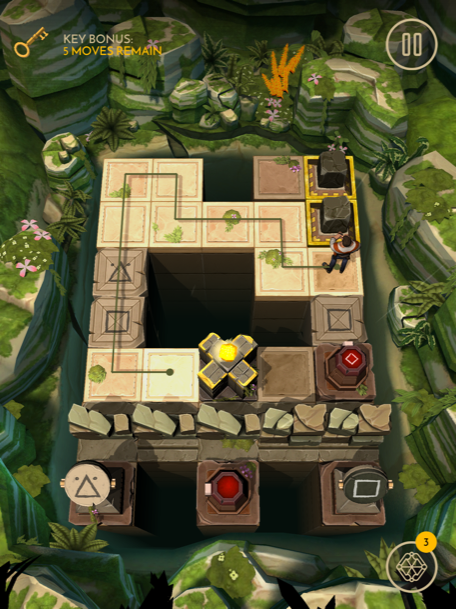### Level 29

First move here.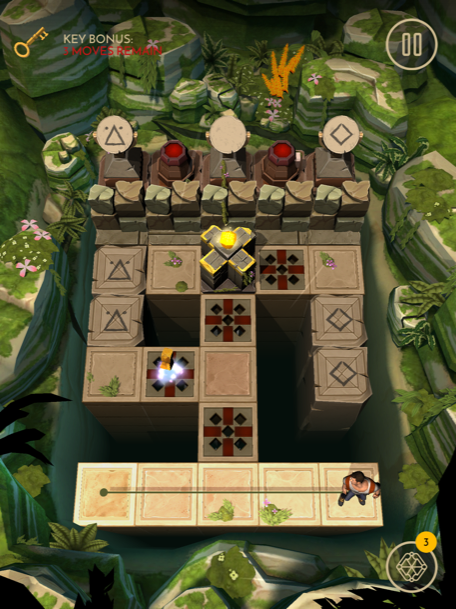Now move here.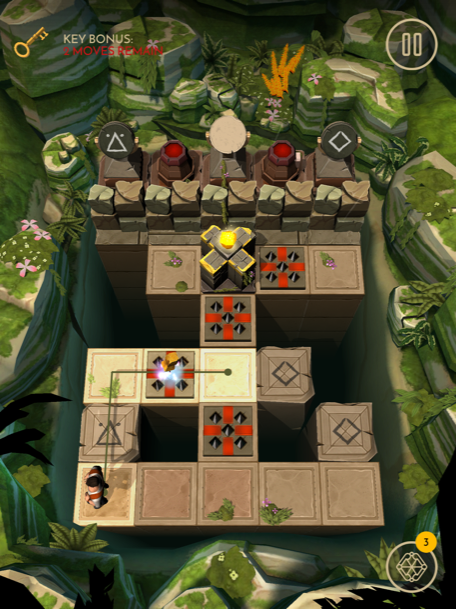Now move here and pick up the treasure.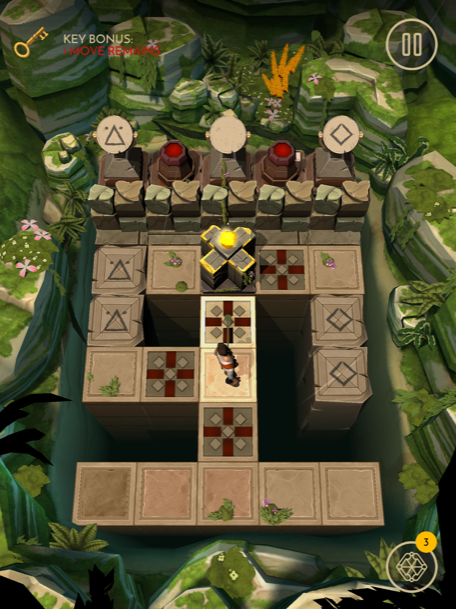### Level 30

First move here and push the switch.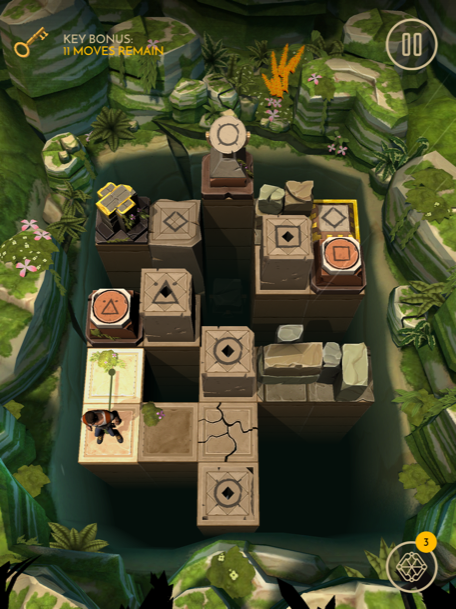Shoot the bomb and the plate, then move here.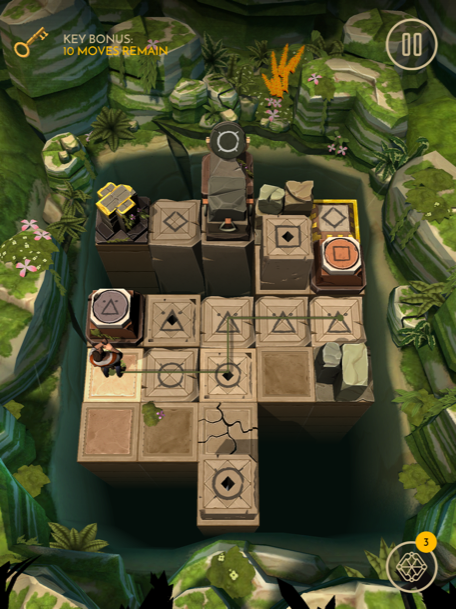Push the switch, shoot the bomb, then move here.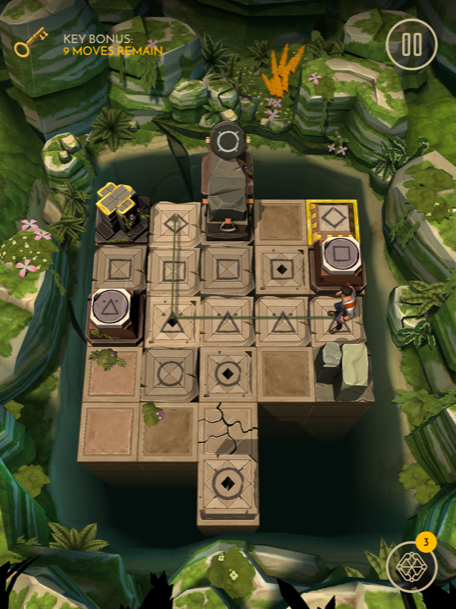Tap the block and push it here.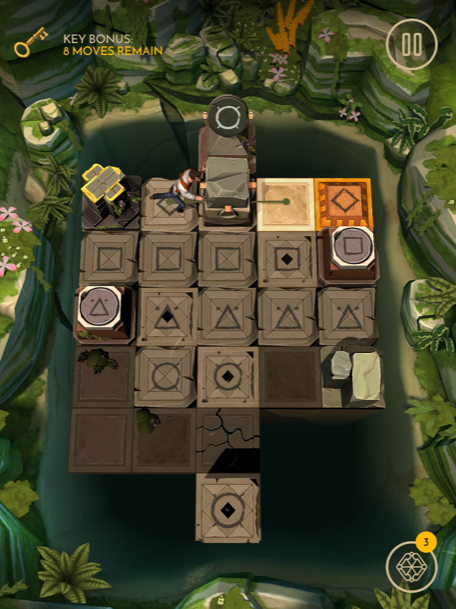Now follow this path and grab the treasure.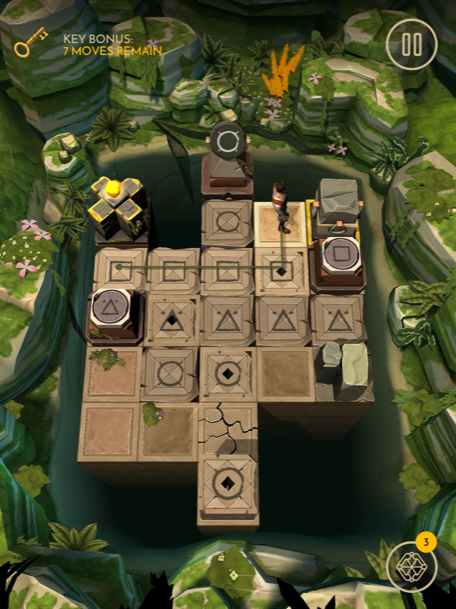Levels 1-10
Levels 11-20
Levels 31-40